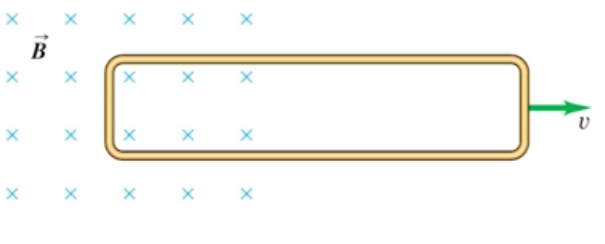Need Help?

Subscribe to Physics 2

###### \${selected_topic_name}
• Notes

$\begin{array}{l}{\text { A rectangular loop of wire with dimensions } 1.50 \mathrm{cm} \text { by }} \\ {8.00 \mathrm{cm} \text { and resistance } R=0.600 \Omega \text { is being pulled to the right out }} \\ {\text { of a region of uniform magnetic field. The magnetic field has mag- - }} \\ {\text { nitude } B=3.50 \mathrm{T} \text { and is directed into the plane of Fig. } \mathrm{E} 29.22 . \text { At }}\end{array}$$\varepsilon=V B L$

$I=\frac{\varepsilon}{R}$

$F_{B}=I L B$

$I=\frac{\varepsilon}{R}=\frac{B L V}{R}$$\longrightarrow F_{B}=\frac{B^{2} L^{2} V}{R}$

$∴ F_{B}=\frac{(3.5)^{2}(0.015)^{2}(3)}{0.6}=0.0138 \mathrm{N}$

$\begin{array}{l}{\text { How fast (in } \mathrm{m} / \mathrm{s} \text { and mph) would a } 5.00 \text { -cm copper bar have to }} \\ {\text { move at right angles to a } 0.650-\mathrm{T} \text { magnetic field to generate } 1.50 \mathrm{V}} \\ {\text { (the same as a AA battery) across its ends? Does this seem like a }} \\ {\text { practical way to generate electricity? }}\end{array}$

$\varepsilon=V B L$

$\varepsilon=V B L$$,\quad \mathrm{l}_\text { mph }=0.447 \mathrm{m} / \mathrm{s}$

$V=\frac{\varepsilon}{B L}$$=\frac{1.5 \mathrm{V}}{0.65 T*5*10^{-2} \mathrm{m}}$

$=103 \mathrm{mph}$

$\rightarrow \text { not }$$\text { practical }$